# Rolling resistance relation with speed

Hi! Well, I'm programming a vehicle's physics, and I have trouble finding a way to calculate Rolling resistance, though i searched a lot. I already have the Traction Force and the Drag Force, but now I need Rolling Resistance. The best thing i found is that Froll = Croll * P, where Croll is the coefficient of rolling and P is the weight of the vehicle, in Newtons. I found info and I made a table for the coefficient being, for example, 0.011 for concrete roads, 0.3 for sand etc, and I have 3 rows for 3 types of wires. The problem, I cannot find how to fit Velocity in the formula.

When going for a 0.011 coefficient the simulation seems to work, but when i choose 0.3, Rolling resistance becomes greater than Traction one, and the vehicle runs backwards before even starting to accelerate. Rolling resistance should start at 0, and increase until the final value as the speed increases, or something like that, but i cannot find any data! I even found people saying that the rolling resistance is 0, and then it jumps to the value after a certain speed threshold, but that sounds weird to me...

wrobel
rolling resistance it is rather a torque=const N, where N is a normal component of ground's reaction

rolling resistance it is rather a torque=const N, where N is a normal component of ground's reaction

Thanks! Torque (N*m) = C * P (N)? That has sense, cause I also found parts talking about the coefficient with the unit m, instead of no unit. But then what is that coefficient, and how do we get the Rolling resistance from that formula?

Now that you talk about Torque, I remember this, I found in wikipedia:

The driving torque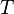to overcome rolling resistance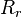and maintain steady speed on level ground (with no air resistance) can be calculated by: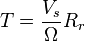where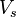is the linear speed of the body (at the axle), and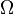its rotational speed.
It is noteworthy that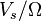is usually not equal to the radius of the rolling body.
V (m/s) / w (rad/s) gives m, so at left, Torque is N*m, at right, we have m*N. So we can calculate the rolling resistance by doing Torque*w / v? So all the info I found, the table with all the coefficients with every type of tyre and every type of road, what's the purpose, if it seems we don't need them?

One thing I dont get now, is that the tyre and the TYPE OF ROAD does not matter in this formula... it should, right?

Last edited:
CWatters
Homework Helper
Gold Member
When going for a 0.011 coefficient the simulation seems to work, but when i choose 0.3, Rolling resistance becomes greater than Traction one, and the vehicle runs backwards before even starting to accelerate.

It appears you have assumed that rolling resistance is a constant force that always acts rearwards. It actually depends on the direction the car is moving. If the car is moving backwards then rolling resistance acts forwards.

It appears you have assumed that rolling resistance is a constant force that always acts rearwards. It actually depends on the direction the car is moving. If the car is moving backwards then rolling resistance acts forwards.

Hmmm... indeed, true, if I go backwards it should go forwards. But it still would fail, cause i don't know how calculate it from speed. So, being at speed = 0, rolling resistance can be 0, but when i start moving... what's rolling resistance? What's the formula to relate it with speed or some other parameters? And does it take into account tires and material of the ground, like the formula i found BUT had not speed into account?

Right now, when i start moving with, let's say, speed = 1 km/h, it instantly go backwards because i calculate rolling speed from the constant and the weight, and it's higher than traction speed...

CWatters
Homework Helper
Gold Member
https://en.wikipedia.org/wiki/Rolling_resistance#Rolling_resistance_coefficient

Rolling resistance is normally modelled as a constant force that is independent of speed. Think of it as a maximum force that varies with the applied force. The algorithm you want is something like this..

IF the applied force > rolling resistance THEN net force = applied force - rolling resistance.
IF the applied force <= rolling resistance THEN net force = zero (eg the rolling resistance is equal to the applied force but opposite sign).

Aside: Are you familiar with static friction? The maximum value of static friction = μN. However if the object doesn't move the friction force is actually equal to the applied force. So if the applied force is zero then static friction is zero. Same with rolling resistance.

Daaamn! That has sense! So the vehicle won't start moving until it can deliver enough force to win against the rolling resistance! (not taking into account drag, cause it's 0 cause speed = 0). I was looking it from a bad point of view... if that's true, then i think it's solver. I'll program it for now, but i'm not sure if the other parts are right (I'm having trouble with gears/transmission) so i cannot say something, but in theory it seems great, and the research i did was fine (based in car weight * coefficient based in tyre and road material) but i did not know how to use it. Lot of thanks!

EDIT: okay, i realised something weird... the tractive force:

double wWheel = speed / wheelRadius
double rpm = min( max( minRPM, wWheel * gear * diff * 60.0 / 2.0*M_PI ), maxRPM );
double torque = scGetTorqueFromRPM( rpm );
double forceTract = torque * gear * diff * efficiency / wheelRadius

So i calculate the force from the torque, the torque from the RPM and the RPM comes from the speed. It worked because RPM has a minimum, so it starts moving but... what happens if the starting RPM (minimum) is not enough to win rolling resistance? Speed will never increase (cause forces will be zero) and without speed, i cannot get MORE RPM to win the resistance.

Does it mean i have to find ANOTHER WAY to calculate RPM other than with speed, so RPM can increase, even at speed=0, and increase, until the force can win the resistance and it can start moving? EDIT2: For now im just increasing RPM for random, not calculating it

Last edited:
jack action
Gold Member
what happens if the starting RPM (minimum) is not enough to win rolling resistance?
The clutch slips or the engine stalls.

As for rolling resistance, it is actually a moment created by the deformation of the wheel, rather than a force. When rotation is initiated, the normal reaction force is moved towards the front of the wheel and the misalignment between the reaction force and the normal load (which is still at the center of the wheel) creates the moment. As the torque increases, the misalignment increases until it reaches its final value.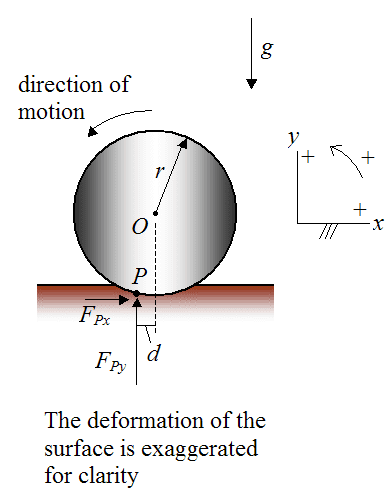When you see a unit of length associated with the rolling resistance coefficient, it corresponds to the length d in the previous figure. The rolling resistance coefficient is then equal to $\frac{d}{r}$ (no units).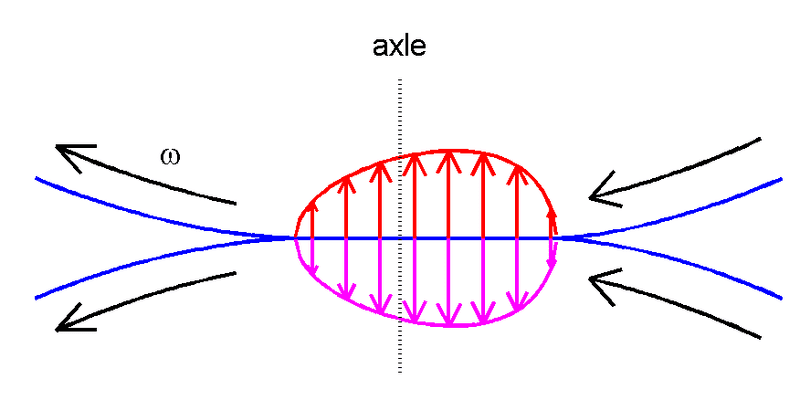This main principle is illustrated in the figure of the rolling cylinders. If two equal cylinders are pressed together then the contact surface is flat. In the absence of surface friction, contact stresses are normal (i.e. perpendicular) to the contact surface. Consider a particle that enters the contact area at the right side, travels through the contact patch and leaves at the left side. Initially its vertical deformation is increasing, which is resisted by the hysteresis effect. Therefore an additional pressure is generated to avoid interpenetration of the two surfaces. Later its vertical deformation is decreasing. This is again resisted by the hysteresis effect. In this case this decreases the pressure that is needed to keep the two bodies separate.

The resulting pressure distribution is asymmetrical and is shifted to the right. The line of action of the (aggregate) vertical force no longer passes through the centers of the cylinders. This means that a moment occurs that tends to retard the rolling motion.

source: https://en.wikipedia.org/wiki/Rolling_resistance#Primary_cause

CWatters